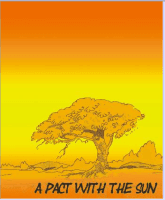Courses

# Geometry Notes - Practical Geometry Class 6 Notes | EduRev

## Class 6: Geometry Notes - Practical Geometry Class 6 Notes | EduRev

The document Geometry Notes - Practical Geometry Class 6 Notes | EduRev is a part of Class 6 category.
All you need of Class 6 at this link: Class 6
``` Page 1

Geometry
Point
Line
Line segment
Ray
Plane
Parallel lines
Intersecting lines
Angles
Point
Line
Line segment
Ray
Plane
Parallel lines
Intersecting lines
Angles
Page 2

Geometry
Point
Line
Line segment
Ray
Plane
Parallel lines
Intersecting lines
Angles
Point
Line
Line segment
Ray
Plane
Parallel lines
Intersecting lines
Angles
Geometry
Point
An exact location in
space
Line
An endless collection
of points along a
straight path
Line segment
Part of a line that has
two endpoints
.A
Point
An exact location in
space
Line
An endless collection
of points along a
straight path
Line segment
Part of a line that has
two endpoints
.A
Page 3

Geometry
Point
Line
Line segment
Ray
Plane
Parallel lines
Intersecting lines
Angles
Point
Line
Line segment
Ray
Plane
Parallel lines
Intersecting lines
Angles
Geometry
Point
An exact location in
space
Line
An endless collection
of points along a
straight path
Line segment
Part of a line that has
two endpoints
.A
Point
An exact location in
space
Line
An endless collection
of points along a
straight path
Line segment
Part of a line that has
two endpoints
.A
Geometry
Ray
Part of a line that has
one endpoint and
extends endlessly in
the other direction
Plane
An endless, flat surface
that is named by any
three points not on the
same line.
Ray
Part of a line that has
one endpoint and
extends endlessly in
the other direction
Plane
An endless, flat surface
that is named by any
three points not on the
same line.
.A
.B
.C
Page 4

Geometry
Point
Line
Line segment
Ray
Plane
Parallel lines
Intersecting lines
Angles
Point
Line
Line segment
Ray
Plane
Parallel lines
Intersecting lines
Angles
Geometry
Point
An exact location in
space
Line
An endless collection
of points along a
straight path
Line segment
Part of a line that has
two endpoints
.A
Point
An exact location in
space
Line
An endless collection
of points along a
straight path
Line segment
Part of a line that has
two endpoints
.A
Geometry
Ray
Part of a line that has
one endpoint and
extends endlessly in
the other direction
Plane
An endless, flat surface
that is named by any
three points not on the
same line.
Ray
Part of a line that has
one endpoint and
extends endlessly in
the other direction
Plane
An endless, flat surface
that is named by any
three points not on the
same line.
.A
.B
.C
Geometry
Parallel Lines
Lines do not intersect
but are in the same
plane
Intersecting Lines
Lines meet at one point
Perpendicular Lines
Lines form a right
angle
Parallel Lines
Lines do not intersect
but are in the same
plane
Intersecting Lines
Lines meet at one point
Perpendicular Lines
Lines form a right
angle
Page 5

Geometry
Point
Line
Line segment
Ray
Plane
Parallel lines
Intersecting lines
Angles
Point
Line
Line segment
Ray
Plane
Parallel lines
Intersecting lines
Angles
Geometry
Point
An exact location in
space
Line
An endless collection
of points along a
straight path
Line segment
Part of a line that has
two endpoints
.A
Point
An exact location in
space
Line
An endless collection
of points along a
straight path
Line segment
Part of a line that has
two endpoints
.A
Geometry
Ray
Part of a line that has
one endpoint and
extends endlessly in
the other direction
Plane
An endless, flat surface
that is named by any
three points not on the
same line.
Ray
Part of a line that has
one endpoint and
extends endlessly in
the other direction
Plane
An endless, flat surface
that is named by any
three points not on the
same line.
.A
.B
.C
Geometry
Parallel Lines
Lines do not intersect
but are in the same
plane
Intersecting Lines
Lines meet at one point
Perpendicular Lines
Lines form a right
angle
Parallel Lines
Lines do not intersect
but are in the same
plane
Intersecting Lines
Lines meet at one point
Perpendicular Lines
Lines form a right
angle
Angles
An angle is formed when two rays have the
same endpoint.
This endpoint is called the vertex.
The two rays that form the angle are called
sides.
An angle is formed when two rays have the
same endpoint.
This endpoint is called the vertex.
The two rays that form the angle are called
sides.
```Use Code STAYHOME200 and get INR 200 additional OFF Use Coupon Code
All Tests, Videos & Notes of Class 6: Class 6

### Top Courses for Class 6### Top Courses for Class 6Track your progress, build streaks, highlight & save important lessons and more!

,

,

,

,

,

,

,

,

,

,

,

,

,

,

,

,

,

,

,

,

,

;